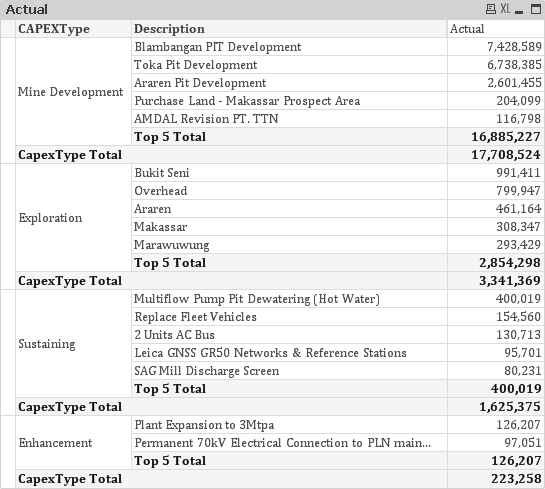QlikView App Dev

Discussion Board for collaboration related to QlikView App Development.

Announcements
QlikWorld 2022, LIVE in Denver CO., May 16-19, 2022. REGISTER NOW TO RECEIVE EARLY BIRD PRICING
cancel
Showing results for
Did you mean:Contributor

[ASK] - Top 5 YTD Value including Total value for Top 5 and Overall Total

Hi Qlik Masters,

I'm back with another question. It seems that each day there are more and more interesting cases during my Qlikview Development.

Without further ado, I present you the case.

Background:

The users expect to see a report that looks like this one.The rules for this report is that every CAPEX Type must have:

1. Top 5 Value - (Top 5 value and sort is based on the YTD value of selected Month, in this case is YTD May 2017)

2. Top 5 Total - (Total of presented Top 5 Value)

3. CAPEX Type Total - (Total YTD May 2017 for every CAPEX Type)

I don't know if this can be done in Qlikview or not. For now the only way that I can think of is to create each object for each CAPEXType, but it contains hard coding and won't be flexible if there are additional CAPEX Type in the future.

Attached with this discussion is the qvw and source file with dummy data.

Your ideas are very much appreciated.

Cheers,

13 RepliesContributor
Author

Hi Sunny,

The confusing part for me is when you put the DIV(INDEX(CONCAT())).

I don't really understand how those three functions work together in the solution. Can you explain a little bit?

Cheers,MVP

That is a way I used to calculate the rank. For more details on this, I have created a post on community. Check this out

Continuous Ranking (no missing Rank)Specialist II

Another way of doing it through the chart presentation is  by unchecking the always Pivot option and taking 2 dimension

Dimension :  CapexType , CapexType, Description

Expression :

pick(Dimensionality() ,

(
SUM({<Year = {"\$(=MAX(Year))"},Month,Date = {"<=\$(=DATE(MAX(Date)))"}>} total<CAPEXType> Actual)) ,

sum( aggrSUM({<Year = {"\$(=MAX(Year))"},Month,Date = {"<=\$(=DATE(MAX(Date)))"}>} Actual)
*
PurgeChar((rank(SUM({<Year = {"\$(=MAX(Year))"},Month,Date = {"<=\$(=DATE(MAX(Date)))"}>}  Actual))<=5),'-') ,CAPEXType,Description)) ,

SUM({<Year = {"\$(=MAX(Year))"},Month,Date = {"<=\$(=DATE(MAX(Date)))"}>}  Actual) *
PurgeChar((rank(SUM({<Year = {"\$(=MAX(Year))"},Month,Date = {"<=\$(=DATE(MAX(Date)))"}>}  Actual))<=5),'-')Contributor
Author

Hi Susant,

Thanks for your proposed solution. I'll use it if the users would like to view the chart simpler.

Cheers,